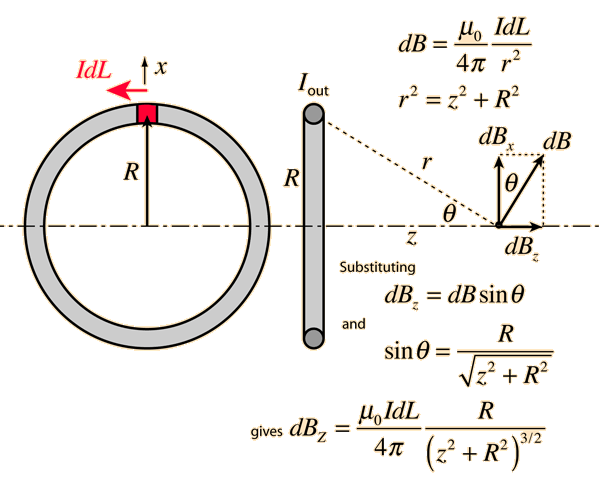Please give the derivation of Magnetic Field at1: Axial Position2: Equatorial Position

• -4The above diagram depicts the magnetic field at axial position

2 At the equatorial line

Let P be a point on the equatorial line of a short bar magnet where flux densitydue to the magnet is to be found out.SN = 2l,Magnetic flux density at p due to N-pole isMagnetic flux density at p due to s-pole isB1 = B2

The resultant magnetic flux density at P isSince the bar magnet is short d >> l, l2 can be neglected as compared to d2.• 9
What are you looking for?